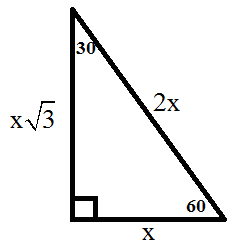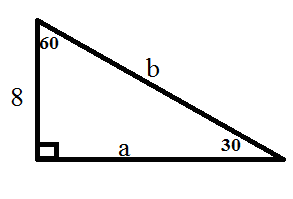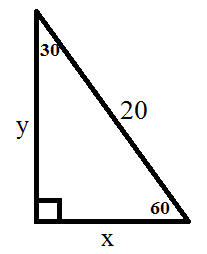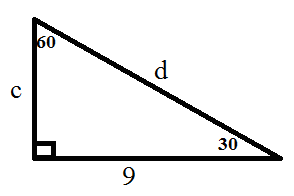# 30-60-90 Triangle: Theorem, Properties & Formula

An error occurred trying to load this video.

Try refreshing the page, or contact customer support.

Coming up next: 45-45-90 Triangle: Theorem, Rules & Formula

### You're on a roll. Keep up the good work!

Replay
Your next lesson will play in 10 seconds
• 0:00 Special Triangles
• 0:46 Qualities Of A…
• 2:10 Example Problems
• 4:48 Lesson Summary

Want to watch this again later?

Timeline
Autoplay
Autoplay
Speed

#### Recommended Lessons and Courses for You

Lesson Transcript
Instructor: Joshua White

Josh has worked as a high school math teacher for seven years and has undergraduate degrees in Applied Mathematics (BS) & Economics/Physics (BA).

This lesson will explore a specific kind of right triangle, the 30-60-90 right triangle, including the relationships that exist between the sides and angles in them.

## Special Triangles

If you've had any experience with geometry, you probably know that there are many different types of triangles. They can be classified by side length (isosceles, scalene, or equilateral) or by angle measurement (acute, obtuse, or right). Additionally, some of these types can be classified even further into smaller groups. This lesson is going to examine one kind of right triangle, which is a triangle that has exactly one right, or 90 degree, angle. This specific kind is a 30-60-90 triangle, which is just a right triangle where the two acute angles are 30 and 60 degrees. Why does this specific triangle have a special name? Let's find out.

## Qualities of a 30-60-90 Triangle

A 30-60-90 triangle is special because of the relationship of its sides. Hopefully, you remember that the hypotenuse in a right triangle is the longest side, which is also directly across from the 90 degree angle. It turns out that in a 30-60-90 triangle, you can find the measure of any of the three sides, simply by knowing the measure of at least one side in the triangle.

The hypotenuse is equal to twice the length of the shorter leg, which is the side across from the 30 degree angle. The longer leg, which is across from the 60 degree angle, is equal to multiplying the shorter leg by the square root of 3. This picture shows this relationship with x representing the shorter leg.Of course, to go in the opposite direction you can divide, instead of multiply, by the appropriate factor. Thus, the relationships can be summarized like this:

Shorter leg ---> Longer Leg: Multiply by square root of 3
Longer leg ---> Shorter Leg: Divide by square root of 3
Shorter Leg ---> Hypotenuse: Multiply by 2
Hypotenuse ---> Shorter Leg: Divide by 2

Notice that the shorter leg serves as a bridge between the other two sides of the triangle. You can get from the longer leg to the hypotenuse, or vice versa, but you first 'pass through' the shorter leg by finding its value. In other words, there is no direct route from the longer leg to the hypotenuse, or vice versa.

## Example Problems

Let's take a look at some examples.

#### Example 1

This is a 30-60-90 triangle with one side length given. Let's find the length of the other two sides, a and b.Since the side you are given, 8, is across from the 30 degree angle, it will be the shorter leg. To find the longer leg, or a, you can simply multiply it by the square root of 3 to get 8 square root 3. To find the hypotenuse, or b, you can simply multiply by the shorter leg by 2. Thus, it will be 8 * 2 = 16.

#### Example 2

Here is a 30-60-90 triangle with one side length given. Let's find the length of the other two sides, x and y.You are given the length of the hypotenuse in this problem. Therefore, you must first find the length of the shorter leg, which is x. You can do this by dividing the hypotenuse, 20, by 2 to get x = 10. Now that you know the value of the shorter leg, you can multiply it by the square root of 3 to find the y. The longer leg will be 10 square root 3.

#### Example 3

Here is a 30-60-90 triangle with one side length given. Let's find the length of the other two sides, c and d.To unlock this lesson you must be a Study.com Member.

### Register to view this lesson

Are you a student or a teacher?

### Unlock Your Education

#### See for yourself why 30 million people use Study.com

##### Become a Study.com member and start learning now.
Back
What teachers are saying about Study.com

### Earning College Credit

Did you know… We have over 160 college courses that prepare you to earn credit by exam that is accepted by over 1,500 colleges and universities. You can test out of the first two years of college and save thousands off your degree. Anyone can earn credit-by-exam regardless of age or education level.